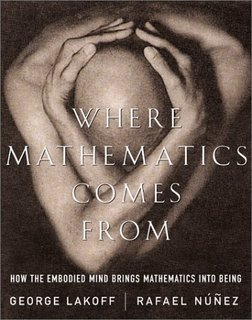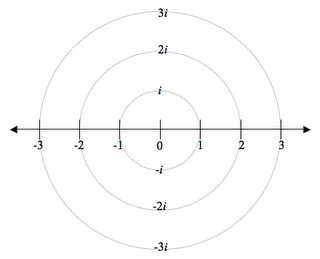## Friday, December 01, 2006

### Next Lesson: Intro to Complex NumbersStill catching up - this was today's lesson...

This lesson's scaffolding is based off of some ideas in the book Where Mathematics Comes From, by George Lakoff. I found this book to be fascinating, as it speaks directly to the question of whether math is a human discovery or a human invention. The book is firmly on the side of invention, explaining how a set of basic "grounding metaphors" create a foundation on which we've built the rest of mathematics. If you're interested in these ideas, I highly recommend reading it. Whether or not you agree with the conclusions, it'll really get you thinking.

In the book, they discuss the metaphor that is used to understand where the imaginary number i comes from. Essentially, you can think of multiplying by -1 on the real number line as a 180 degree rotation. Once you accept this metaphor, then it opens the question: multiplying by what number gives you a 90 degree rotation? If you multiply by that number twice, that means you've rotated 90 degrees twice. Since this is equal to 180 degrees, multiplying by the mystery number twice gives the same result as multiplying by -1. Therefore, the mystery number must be the square root of -1. Whoa. I thought that was an interesting way of looking at it. It allows you to then see why the imaginary axis is perpendicular to the real axis. It also allows you to see why the powers of i follow that 4 number pattern which students need to know. You can go around in a circle, stopping every 90 degrees at an axis. If you start from 1 on the real axis, you'll pass through 1, i, -1, -i, then back to 1. Also, this had an added benefit: one student asked what would happen if you rotate 180 degrees twice. He realized that this would be the same as multiplying by -1 twice. When I pointed out that this rotation would get you back to where you started from, I think his jaw dropped a bit - he may have finally understood a reason that explains why a "negative times a negative is a positive"!So I designed a handout for students to work through these ideas, we discussed them, and I finished with about 20 minutes of lecture to tie it all together and do some exaxmples. I love this lesson, because I feel like it allows for some deep learning, and the mathematics are not that tough, so almost all students can follow it pretty easily.

This lesson will be posted on ILoveMath.

Darren said...

I just introduced i this last week. I like the "rotation" idea!

If you're interested in "math" books, I recommend 2 by former UC Davis professor Sherman Stein--Strength in Numbers, and How The Other Half Thinks. They're written in a clear, conversational style, and the average Joe, not the hardcore mathematician, is the target audience.Anonymous said...

I love this metaphor / way of explaining things. Your diagram might benefit from a vertical axis. And it looks like I am going to need to read Lakoff's book!

Dan Wekselgreene said...

Darren,
I'll check those out. The Lakoff book isn't really targeted to hardcore mathematicians either (otherwise, I wouldn't be able to read it!). It's written by a linguist and a cognitive scientist. I wouldn't call it "conversational", however. :)

MRC,
I thought about putting in a vertical axis to start with, but decided not to. Actually, on the diagram I gave the students, I left off the imaginary numbers too - I just gave them a number line with the circles to guide the rotations. I wanted them to create the vertical axis themselves by using the rotation metaphor. I think it was a good choice - some students thought that a 90 degree rotation would be the same as multiplying by 0, since you land above 0 on the real number line. But then other students realized that you were no longer on the real number line, so maybe it wasn't a real number at all. I actually hadn't thought of it that way myself before our discussion. I think that giving the vertical axis might have stifled some of the initial discussion.

After this piece of it, I gave them an actual complex plane to practice plotting complex numbers. I think I did a better job this year of getting them to understand that a complex number has a real and an imaginary component; graphically, any number on one of the axes is purely real or purely imaginary, and anything in the grid area is a combination of the two, and is therefore complex. I think I should have done a better job of connecting the circle diagram to the complex plane to reinforce where the plane comes from. I'll do this more explicitly when we go back to do the powers of i lesson.

The book has a lot of great stuff in it. At the end, there are a couple of case studies, where they explain how their theories can be used to understand both the number e and the equation e^pi*i + 1 = 0.Anonymous said...

It is very useful, yes, to see multiplication by complex numbers as rotations; since you were also fiddling with matrices and solving linear systems, you might point out that rotation by an angle theta can be seen as a 2x2 matrix:

cos theta | -sin theta
sin theta | cos theta

This is fun stuff to fiddle around with. A good linear algebra book like Axler's "Linear Algebra Done Right" (aimed at undergraduates) or Paul Halmos' "Finite Dimensional Vector Spaces," will give you more fun things about the spatial relationships between complex numbers and linear algebra.

-- xnAnonymous said...

It looks like wikipedia has a graphic on their page for Euler's Identity that's based on the same thing.

I like what you wrote about leaving space for the discovery. I recently had a similar experience where a student showed me a new way to see something familiar.